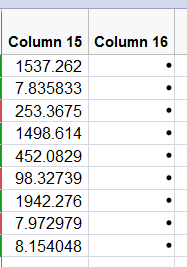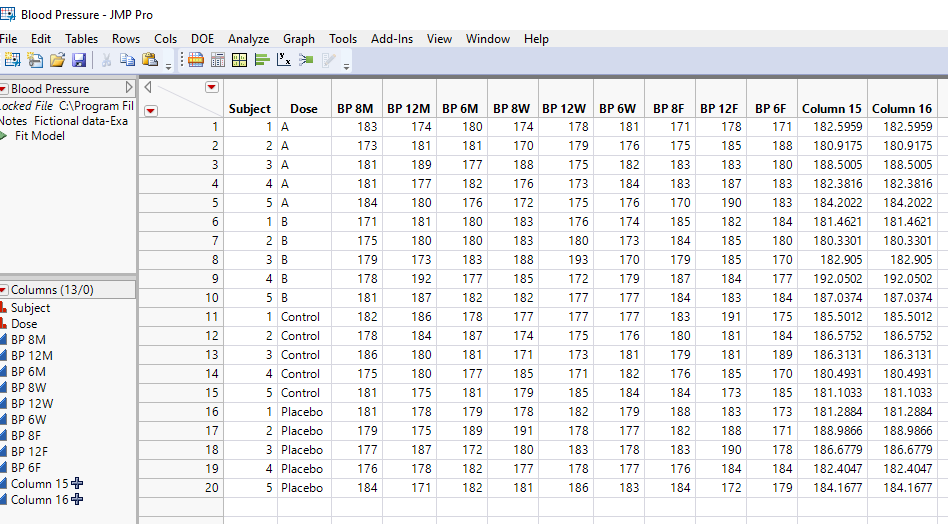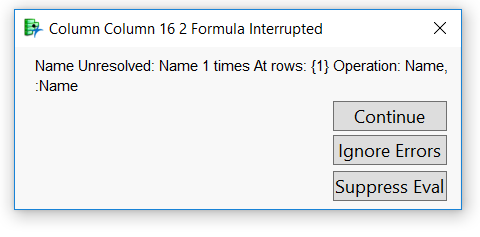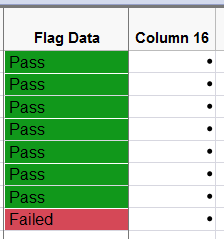The World Statistics Day celebration continues here in the Community. We all need reliable data for sound decision making. Do you have a data source that you trust most? Head over to Discussions to tell us about it.
Choose Language Hide Translation Bar
Highlighted

## Maximum value of several variable columns to specific rows

Hi all,

How to create a formula column to find the maximum of several variable columns to specific rows

``````colList = dt << get column names;colList = remove( colList, 1,2);
Show( colList );

New Column( "Column 15",
Numeric,
Continuous,
Format( "Best", 8 ),
Formula( Maximum(colList), colList,colList ) ),
Set Selected
);``````

8 REPLIES 8
Highlighted

Highlighted

## Re: Maximum value of several variable columns to specific rows

Below is a script that calculates what you want two different ways.  The first, is a correction to your code.  Some "(" had to be moved since they were not in the correct place, and also, the As Column() function had to be added to tell JMP to treat the colList[] values as columns.  While this code works, if you ever quit JMP and then come back into JMP, the formula would not work, because, there would not be a list called "colList" to use in the formula.  So what you really need to use is what I have put in as a new column, "Column 16".  Run the script on your data, and then look at the actual formulas for the two columns, and you will see the difference.

``````Names Default To Here( 1 );
dt = Current Data Table();

colList = dt << get column names;
colList = Remove( colList, 1, 2 );
Show( colList );

New Column( "Column 15",
Numeric,
Continuous,
Format( "Best", 8 ),
Formula(
Max(
As Column( colList ),
As Column( colList ),
As Column( colList )
),
Set Selected
)
);

Eval(
Substitute(
Expr(
New Column( "Column 16",
Numeric,
Continuous,
Format( "Best", 8 ),
Formula(
Max( __col1__, __col2__, __col3__ ),
Set Selected
)
)
),
Expr( __col1__ ), Parse( ":" || Char( colList ) ),
Expr( __col2__ ), Parse( ":" || Char( colList ) ),
Expr( __col3__ ), Parse( ":" || Char( colList ) )
)
);``````
Jim
Highlighted

## Re: Maximum value of several variable columns to specific rows

* The first solution (Colmun 15) it's working good

* The second (Colmun 16) not working very wellHighlighted

## Re: Maximum value of several variable columns to specific rows

Here is my script, with the only change that it is pointing to the the SAMPLE data table "Blood Presure".``````Names Default To Here( 1 );
dt = Open("\$SAMPLE_DATA/Blood Pressure.jmp");

colList = dt << get column names;
colList = Remove( colList, 1, 2 );
Show( colList );

New Column( "Column 15",
Numeric,
Continuous,
Format( "Best", 8 ),
Formula(
Max(
As Column( colList ),
As Column( colList ),
As Column( colList )
),
Set Selected
)
);

Eval(
Substitute(
Expr(
New Column( "Column 16",
Numeric,
Continuous,
Format( "Best", 8 ),
Formula(
Max( __col1__, __col2__, __col3__ ),
Set Selected
)
)
),
Expr( __col1__ ), Parse( ":" || Char( colList ) ),
Expr( __col2__ ), Parse( ":" || Char( colList ) ),
Expr( __col3__ ), Parse( ":" || Char( colList ) )
)
);``````

The issue may be that you have complex column names, that have characters in them that JMP is trying to do calculations on.  If that is the case, you need to change the Substitution to:

``Expr( __col1__ ), Parse( ":Name\!"(" || Char( colList ) || "\!")" ) ``

Of course, change if for all 3 Substitutions

Jim
Highlighted

## Re: Maximum value of several variable columns to specific rows

Sorry, but it's still not working (solution 2).

My coulmns names - Date, format -01/30/2019 .

Thx.Highlighted

## Re: Maximum value of several variable columns to specific rows

Does the sample code that I provided work, just as I have provided it?  It should work without change.

The error statement indicates that you are having a problem with the recognition of the word "Name" . Could you provide a sample of your data, and your script that is failing?

Jim
Highlighted

## Re: Maximum value of several variable columns to specific rows

@txnelson  I am running across a similar issue, but instead of knowing my column length, like op knows his length of 3. How do I modify it so it will do the max of n length?

Highlighted

## Re: Maximum value of several variable columns to specific rows

See the following Discussion here.

Jim
Article Labels

There are no labels assigned to this post.